# [2107.07110] Recurrent Parameter Generators

### Significance

Generating parameters from fixed weight matrix promotes efficiency

### Keypoints

• Propose a method for generating parameters from fixed weight matrix
• Show efficiency and performance of the proposed method by experiments

### Review

#### Background

Recent trend in scaling up neural networks and dataset size have shown to improve model performance. However, these large number of model parameters are not always fully efficient and show high redundancy. Pruning-based model compression methods address this issue and try to remove unnecessary parameters from a trained neural network. This work takes an opposite direction of model compression by generating parameters from fixed weights with maximal efficiency.

#### Keypoints

##### Propose a method for generating parameters from fixed weight matrix

The proposed method is named recurrent parameter generator (RPG).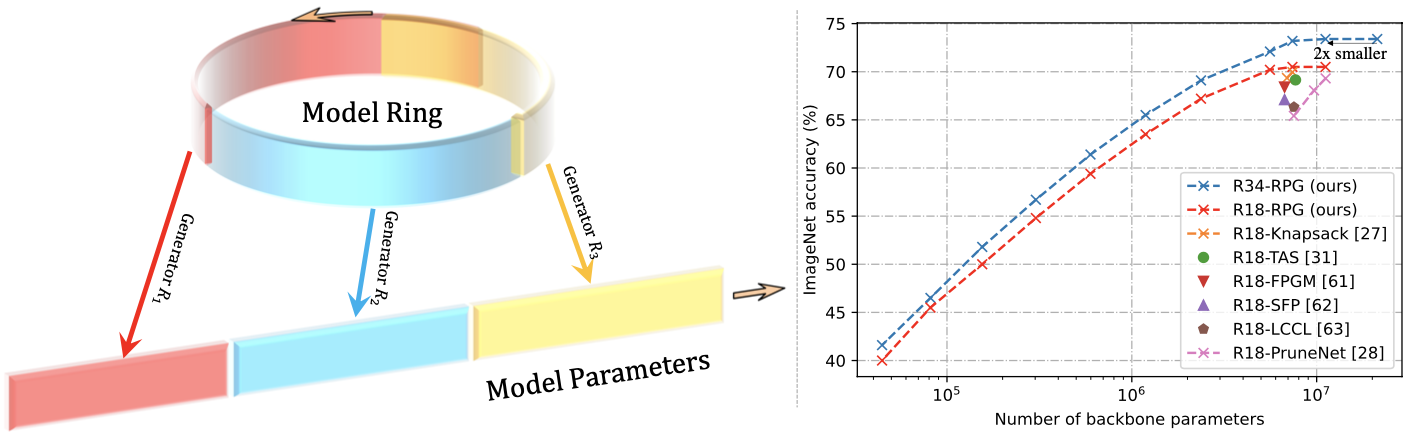Schematic illustration of the proposed method (left), and the performance with respect to number of generated parameters (right)

Let $\mathbf{K}_{1},\mathbf{K}_{2},…,\mathbf{K}_{L}$ be convolution kernels at layer $i \in {1,2,…,L}$. For each convolution layer, the kernel is defined as: \begin{align}\label{eq:rpg} \mathbf{K}_{i} = \mathbf{R}_{i}\cdot \mathbf{W}, i \in {1,2,…,L}, \end{align} where $\mathbf{R}_{i}$ is the generating matrix at layer $i$ and the $\mathbf{W} \in \mathbb{R}^{N}$ is the fixed parameter matrix (vector) with $N$ elements, called the model ring in this work. Given that the size of $\mathbf{W}$ is smaller than the total number of parameters in the target model architecture, the RPG can induce efficiency to the model. It can be easily shown that \eqref{eq:rpg} is differentiable and can be backpropagated with respect to the final loss. The choice for generating matrix $\mathbf{R}$ is based on the destructive weight sharing and even sampling strategy, which the details are referred to the original paper.

One RPG suffices to construct a full model architecture, e.g. ResNet18, while multiple local RPGs can also be used to construct blocks of the full model architecture.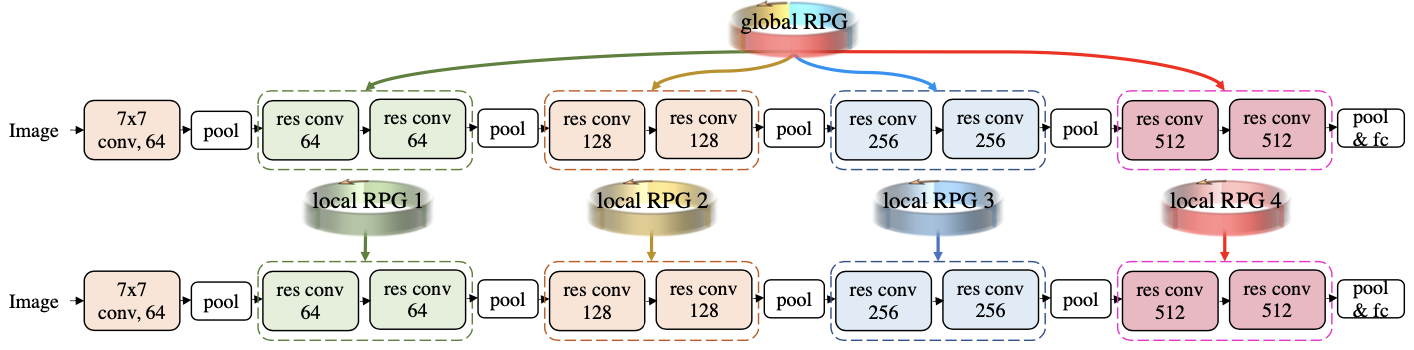Example of global and local approach of RPG for generating ResNet18 parameters

##### Show efficiency and performance of the proposed method by experiments

The proposed RPG is experimented on various tasks including image classification, pose estimation, and multi-task regression.

###### Image classification

Image classification performance is experimented on the CIFAR-10, CIFAR-100, and ImageNet datasets.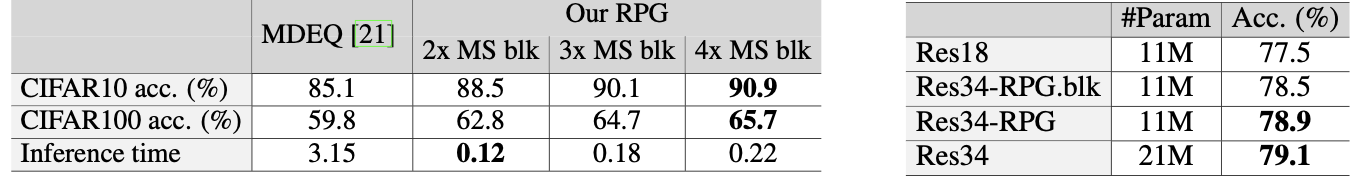Comparison of RPG with baseline method (left). RPG outperforms conventional model architectures with same number of parameters (right) It can be seen that the proposed method outperforms baseline method and conventional model architectures without parameter generation on the CIFAR-10 and CIFAR-100 datasets.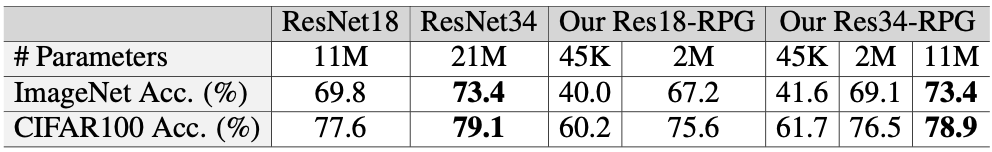Performance of the proposed method on CIFAR-100 and ImageNet dataset This performance gain is also apparent for image classification on the ImageNet dataset. Accuracy and the number of parameters follow power law as in the conventional neural network architectures as can be seen in the right side of the topmost figure.

###### Pose estimation

Pose estimation with RPG is experimented on the MPII human pose dataset. The percentage of correct key-points at 50\% threshold (PCK@0.5) is evaluated for the proposed method and the baselines.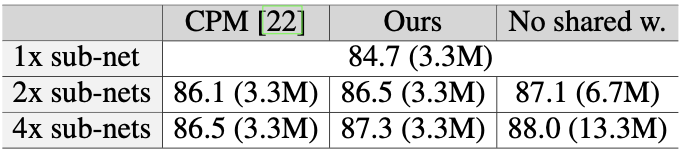Pose estimation performance on the MPII human pose dataset It can be seen that the proposed method outperforms Convolutional pose machine (CPM) which shares all parameters for different sub-networks.

Performance of the multi-task regression is evaluated on the Stanford 3D indoor scene dataset.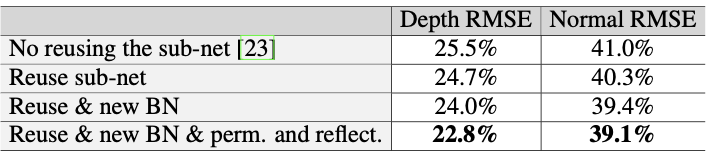Multi-task regression results